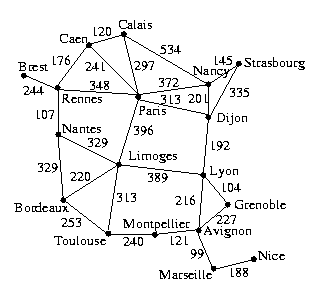Harrison Chapman

Math 301: Homework 11

Due: Friday, December 6, 2019

Exercises:

1. Is the given code a valid Prufer code? Without computing the Extended Prufer code, answer yes or no. If the answer is no, explain why. If the answer is yes, draw the corresponding tree.

1. 4205423

2. 1592016

3. 4444444

1. Draw a connected weighted graph that has at least two different minimal spanning trees.

2. Draw a connected weighted graph which has a unique minimal spanning tree, but in which two edges have the same weight.

1. Find and draw a minimal spanning tree for the graph below.2. What is the name of the algorithm you used in part (a)?